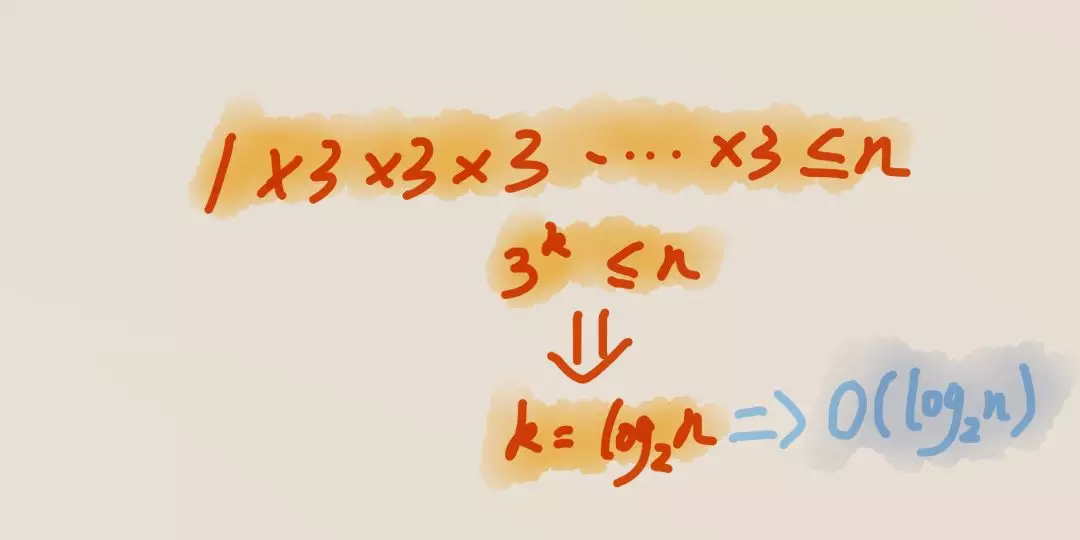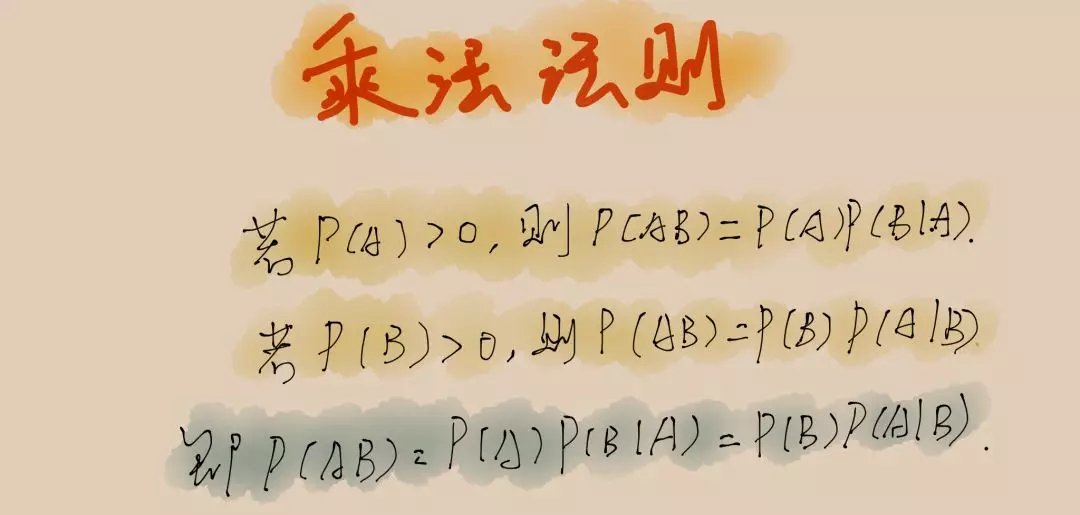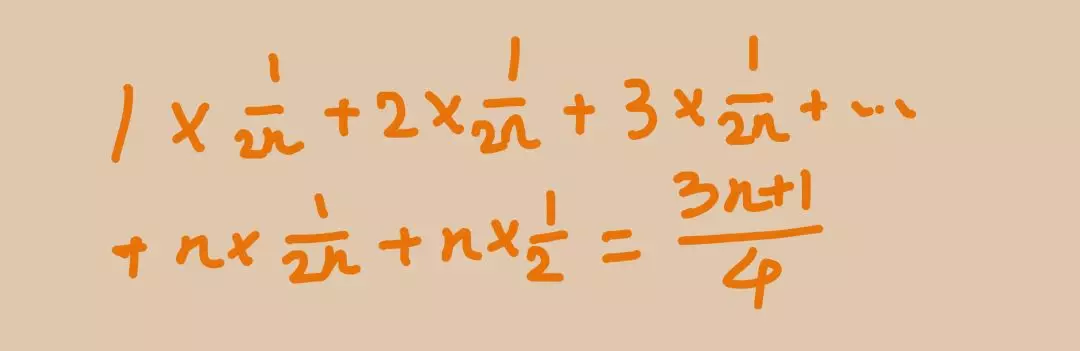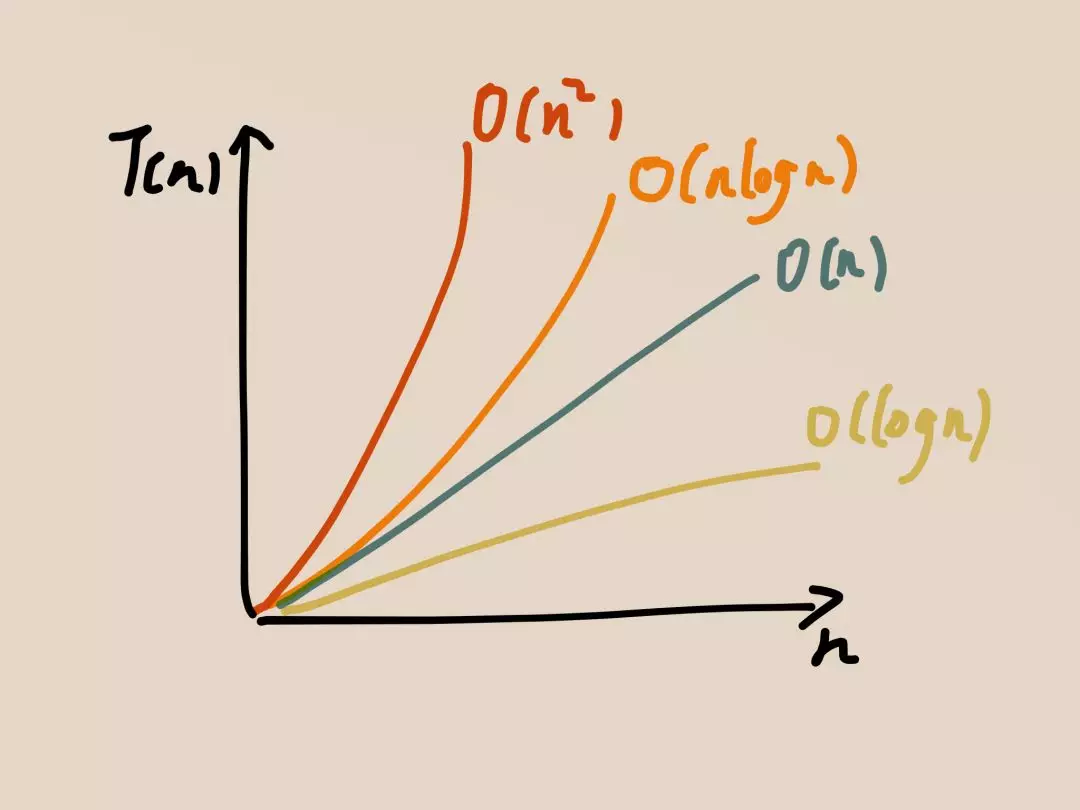# 一文概括复杂度分析• [单段代码看频率]：看代码片段中「循环代码」的时间复杂度。

• [多段代码看最大]：如果多个 for 循环，看「嵌套循环最多」的那段代码的时间复杂度。

• [嵌套代码求乘积]：循环、递归代码，将内外嵌套代码求乘积去时间复杂度。

• [多个规模求加法]:法有两个参数控制两个循环的次数，那么这时就取二者复杂度相加。

----------------------------------❤------------------------------------

## 时间复杂度

1、什么是复杂度？

2、分析的三个方法

Example:

// 求n个数字之和
int xiaolu(int n) {
int sum = 0;
for (int i = 1; i <= n; ++i) {
sum = sum + i;
}
return sum;
}


Example:

int xiaolu(int n) {
int sum = 0;
//循环一
for (int i = 1; i <= 100; j++) {
sum = sum + i;
}
//循环二
for (int j = 1; j <= n; j++) {
sum = sum + i;
}
}


Example:

 for (int j = 1; j <= n; j++) {
for(int i = 1; i <= n; i++)
sum = sum + i;
}


## 空间复杂度

1、什么是空间复杂度？

Example:

 int xiaolu(int n) {
int sum = 0;
//循环一
for (int i = 1; i <= 100; j++) {
sum = sum + i;
}
//循环二
for (int j = 1; j <= n; j++) {
sum = sum + i;
}
}


2、最常见的空间复杂度

O(1)、O(n)、O(n²)。

O(1)

Example:

int i = 1;
int j = 2;
int sum = i + j;


O(logn) | O(nlogn)

「对数阶时间复杂度」，最难分析的一种时间复杂度。

Example:

 i=1;
while (i <= n)  {
i = i * 3;
}O(m+n) | O(mn)*

----------------------------------❤------------------------------------

#### 1、最好、最坏时间复杂度

Example

 //在一个 array 数组中查找一个数据 a 是否存在
for (int i = 1; i < n; i++) {
if (array[i] == a) {
return i;
}
}


1、最好情况就是数组的第一个就是我们要查找的数据，上边代码之执行一遍就可以，这种情况下的时间复杂度为最好时间复杂度，为 O(1)。

2、最坏的情况就是数组的最后一个才是我们要查找的数据，需要循环遍历 n 遍数组，也就对应最坏的时间复杂度为 O(n) 。

#### 2、平均时间复杂度#### 3、均摊时间复杂度===========我是华丽的分割线===========02-151419
12-17118
08-29228
10-18272
11-184725
06-23642
07-301625
12-05839
07-291万+
07-092066
12-27245
04-27428
08-29118
12-252632
12-201万+
06-01444
04-124667
12-28128

### “相关推荐”对你有帮助么？

•非常没帮助
•没帮助
•一般
•有帮助
•非常有帮助被折叠的  条评论 为什么被折叠?到【灌水乐园】发言Leon_George

¥2 ¥4 ¥6 ¥10 ¥20余额支付 (余额：-- )扫码支付获取中扫码支付点击重新获取扫码支付1.余额是钱包充值的虚拟货币，按照1:1的比例进行支付金额的抵扣。
2.余额无法直接购买下载，可以购买VIP、C币套餐、付费专栏及课程。余额充值